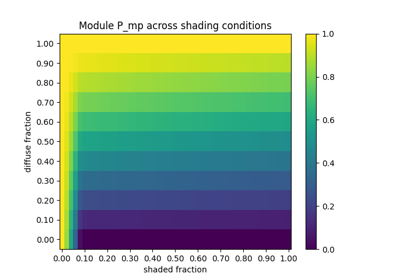# pvlib.singlediode.bishop88#

pvlib.singlediode.bishop88(diode_voltage, photocurrent, saturation_current, resistance_series, resistance_shunt, nNsVth, d2mutau=0, NsVbi=inf, breakdown_factor=0.0, breakdown_voltage=- 5.5, breakdown_exp=3.28, gradients=False)[source]#

Explicit calculation of points on the IV curve described by the single diode equation. Values are calculated as described in 1.

The single diode equation with recombination current and reverse bias breakdown is

$I = I_{L} - I_{0} \left (\exp \frac{V_{d}}{nN_{s}V_{th}} - 1 \right ) - \frac{V_{d}}{R_{sh}} - \frac{I_{L} \frac{d^{2}}{\mu \tau}}{N_{s} V_{bi} - V_{d}} - a \frac{V_{d}}{R_{sh}} \left (1 - \frac{V_{d}}{V_{br}} \right )^{-m}$

The input diode_voltage must be $$V + I R_{s}$$.

Warning

• Usage of d2mutau is required with PVSyst coefficients for cadmium-telluride (CdTe) and amorphous-silicon (a:Si) PV modules only.

• Do not use d2mutau with CEC coefficients.

Parameters
• diode_voltage (numeric) – diode voltage $$V_d$$ [V]

• photocurrent (numeric) – photo-generated current $$I_{L}$$ [A]

• saturation_current (numeric) – diode reverse saturation current $$I_{0}$$ [A]

• resistance_series (numeric) – series resistance $$R_{s}$$ [ohms]

• resistance_shunt (numeric) – shunt resistance $$R_{sh}$$ [ohms]

• nNsVth (numeric) – product of thermal voltage $$V_{th}$$ [V], diode ideality factor $$n$$, and number of series cells $$N_{s}$$ [V]

• d2mutau (numeric, default 0) – PVsyst parameter for cadmium-telluride (CdTe) and amorphous-silicon (a-Si) modules that accounts for recombination current in the intrinsic layer. The value is the ratio of intrinsic layer thickness squared $$d^2$$ to the diffusion length of charge carriers $$\mu \tau$$. [V]

• NsVbi (numeric, default np.inf) – PVsyst parameter for cadmium-telluride (CdTe) and amorphous-silicon (a-Si) modules that is the product of the PV module number of series cells $$N_{s}$$ and the builtin voltage $$V_{bi}$$ of the intrinsic layer. [V].

• breakdown_factor (float, default 0) – fraction of ohmic current involved in avalanche breakdown $$a$$. Default of 0 excludes the reverse bias term from the model. [unitless]

• breakdown_voltage (float, default -5.5) – reverse breakdown voltage of the photovoltaic junction $$V_{br}$$ [V]

• breakdown_exp (float, default 3.28) – avalanche breakdown exponent $$m$$ [unitless]

• gradients (bool) – False returns only I, V, and P. True also returns gradients

Returns

tuple – currents [A], voltages [V], power [W], and optionally $$\frac{dI}{dV_d}$$, $$\frac{dV}{dV_d}$$, $$\frac{dI}{dV}$$, $$\frac{dP}{dV}$$, and $$\frac{d^2 P}{dV dV_d}$$

Notes

The PVSyst thin-film recombination losses parameters d2mutau and NsVbi should only be applied to cadmium-telluride (CdTe) and amorphous- silicon (a-Si) PV modules, 2, 3. The builtin voltage $$V_{bi}$$ should account for all junctions. For example: tandem and triple junction cells would have builtin voltages of 1.8[V] and 2.7[V] respectively, based on the default of 0.9[V] for a single junction. The parameter NsVbi should only account for the number of series cells in a single parallel sub-string if the module has cells in parallel greater than 1.

References

1

“Computer simulation of the effects of electrical mismatches in photovoltaic cell interconnection circuits” JW Bishop, Solar Cell (1988) DOI: 10.1016/0379-6787(88)90059-2

2

“Improved equivalent circuit and Analytical Model for Amorphous Silicon Solar Cells and Modules.” J. Mertens, et al., IEEE Transactions on Electron Devices, Vol 45, No 2, Feb 1998. DOI: 10.1109/16.658676

3

“Performance assessment of a simulation model for PV modules of any available technology”, André Mermoud and Thibault Lejeune, 25th EUPVSEC, 2010 DOI: 10.4229/25thEUPVSEC2010-4BV.1.114

## Examples using pvlib.singlediode.bishop88#Calculating power loss from partial module shading

Calculating power loss from partial module shading